### Home > CALC > Chapter 3 > Lesson 3.4.1 > Problem3-151

3-151.
1. State the domain and the range of the functions below. Homework Help ✎

1.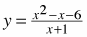2.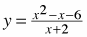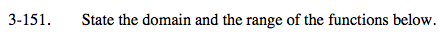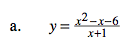Factor first.

This function has a vertical asymptote at x = −1. How does that affect range?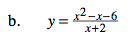Factor first.

This function has a hole at x = −2. It's end behavior is y = x − 3, which can help you find the y-value of the hole.